# Python | Pandas TimedeltaIndex.append()

Python is a great language for doing data analysis, primarily because of the fantastic ecosystem of data-centric python packages. Pandas is one of those packages and makes importing and analyzing data much easier.

Pandas` TimedeltaIndex.append()` function appends a collection of index options together. More than one index object can be appended at a time by passing the indexes as a python list or tuple.

Syntax : TimedeltaIndex.append(other)

Parameters :
other : Index or list/tuple of indices

Return : appended : Index

Example #1: Use `TimedeltaIndex.append()` function to append a TimedeltaIndex object at the end of the given object.

 `# importing pandas as pd ` `import` `pandas as pd ` ` `  `# Create the first TimedeltaIndex object ` `tidx1 ``=` `pd.TimedeltaIndex(start ``=``'1 days 02:00:12.001124'``, periods ``=` `5``, ` `                                              ``freq ``=``'N'``, name ``=``'Koala'``) ` ` `  `# Create the second TimedeltaIndex object ` `tidx2 ``=` `pd.TimedeltaIndex(data ``=``[``'-1 days 2 min 3us 10ns'``, ` `                                 ``'1 days 06:05:01.000030'``,  ` `                                 ``'-1 days + 23:59:59.999999'``]) ` ` `  `# Print the first TimedeltaIndex object ` `print``(tidx1) ` ` `  `# Print the second TimedeltaIndex object ` `print``(tidx2) `

Output :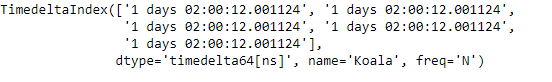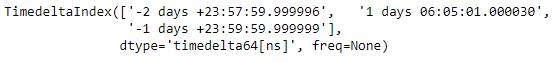Now we will append tidx2 at the end of tidx1.

 `# append tidx2 at the end of tidx1 ` `tidx1.append(tidx2) `

Output :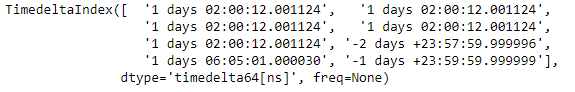As we can see in the output, the `TimedeltaIndex.append()` function has appended tidx2 at the end of tidx1.

Example #2: Use `TimedeltaIndex.append()` function to append a list of TimedeltaIndex object at the end of the given object.

 `# importing pandas as pd ` `import` `pandas as pd ` ` `  `# Create the first TimedeltaIndex object ` `tidx1 ``=` `pd.TimedeltaIndex(start ``=``'1 days 02:00:12.001124'``, periods ``=` `5``, ` `                                              ``freq ``=``'N'``, name ``=``'Koala'``) ` ` `  `# Create the second TimedeltaIndex object ` `tidx2 ``=` `pd.TimedeltaIndex(data ``=``[``'-1 days 2 min 3us 10ns'``, ` `                                 ``'1 days 06:05:01.000030'``, ` `                                 ``'-1 days + 23:59:59.999999'``]) ` ` `  `# Create the third TimedeltaIndex object ` `tidx3 ``=` `pd.TimedeltaIndex(data ``=``[``'-3 days 02:10:00'``, ` `                                 ``'1 days 06:05:01.000030'``, ` `                                 ``'1 days 02:00:00'``], name ``=``'MyObjejct'``) ` ` `  `# Print the first TimedeltaIndex object ` `print``(tidx1) ` ` `  `# Print the second TimedeltaIndex object ` `print``(tidx2) ` ` `  `# Print the third TimedeltaIndex object ` `print``(tidx3) `

Output :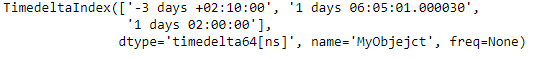Now we will append tidx2 and tidx3 at the end of tidx1.

 `# append tidx2 and tidx3 at the end of tidx1 ` `tidx1.append([tidx2, tidx3]) `

Output :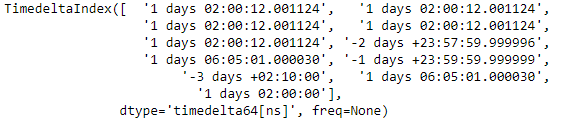As we can see in the output, the `TimedeltaIndex.append()` function has appended tidx2 and tidx3 at the end of tidx1.

My Personal Notes arrow_drop_upCheck out this Author's contributed articles.

If you like GeeksforGeeks and would like to contribute, you can also write an article using contribute.geeksforgeeks.org or mail your article to contribute@geeksforgeeks.org. See your article appearing on the GeeksforGeeks main page and help other Geeks.

Please Improve this article if you find anything incorrect by clicking on the "Improve Article" button below.

Article Tags :

Be the First to upvote.

Please write to us at contribute@geeksforgeeks.org to report any issue with the above content.